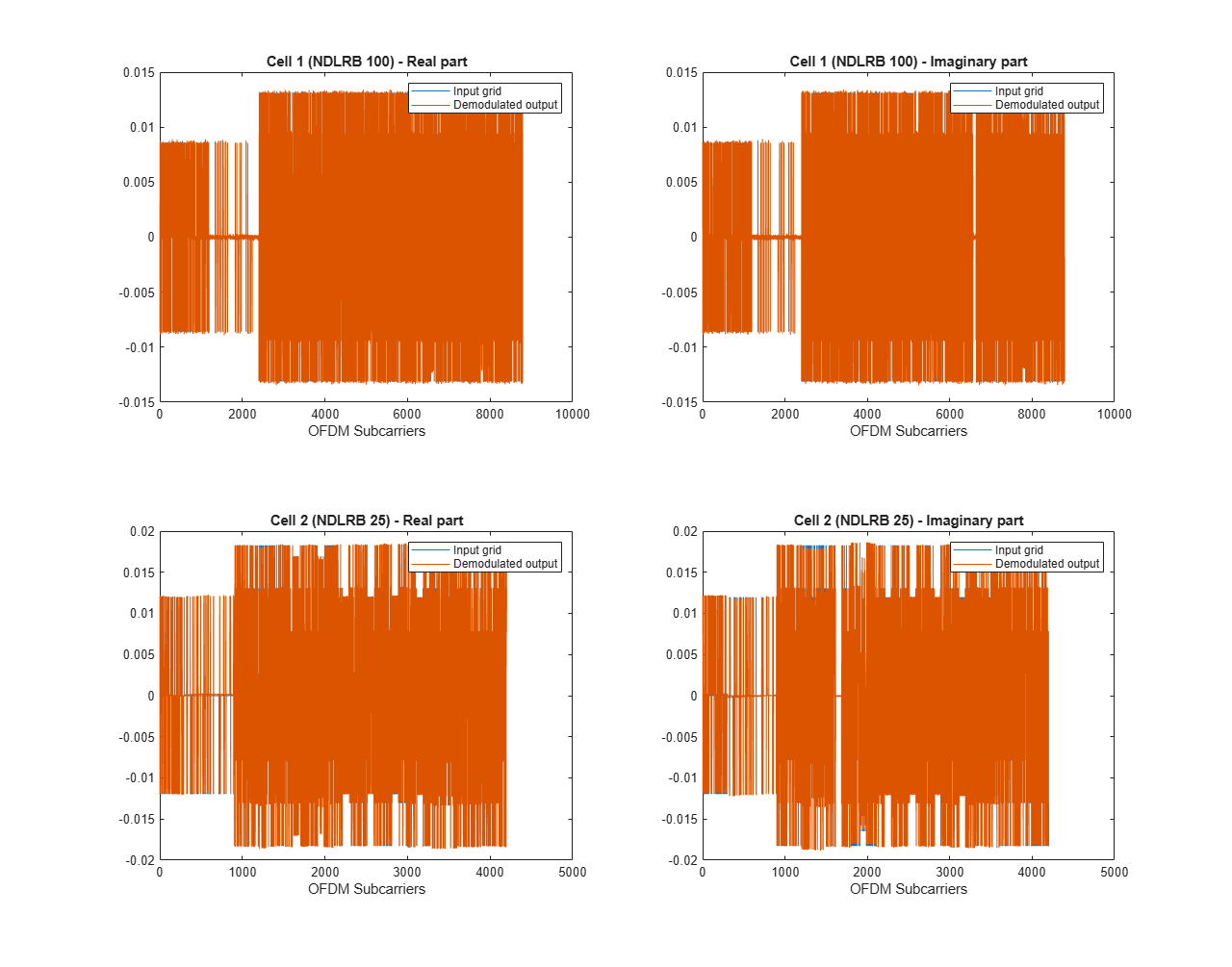# Reset and Restart LTE OFDM Demodulation

This example shows how to recover the LTE OFDM Demodulator block from an unfinished LTE cell. The input data is truncated to simulate the loss of a signal or a reset from the upstream parts of the receiver. The example model uses the reset signal to clear the internal state counters of the LTE OFDM Demodulator block and then restart calculations on the next cell. In this example, the Input data sample rate parameter of LTE OFDM Demodulator is set to Use maximum input data sample rate. So, the base sampling rate of the block is 30.72 MHz.

Generate two input LTE OFDM cells that use different NDLRBs or different types of cyclic prefix. Upsample both waveforms to the base sampling rate of 30.72 MHz.

```% ------------------------------------------------------------ % NDLRB | Reference Channel % ------------------------------------------------------------ % 6 | R.4 % 15 | R.5 % 25 | R.6 % 50 | R.7 % 75 | R.8 % 100 | R.9 % ------------------------------------------------------------ enb1 = lteRMCDL('R.9'); enb1.TotSubframes = 1; enb1.CyclicPrefix = 'Normal'; % or 'Extended' [waveform1,grid1,info1] = lteRMCDLTool(enb1,[1;0;0;1]); enb2 = lteRMCDL('R.6'); enb2.TotSubframes = 1; enb2.CyclicPrefix = 'Normal'; % or 'Extended' [waveform2,grid2,info2] = lteRMCDLTool(enb2,[1;0;0;1]); FsRx = 30.72e6; tx1 = resample(waveform1,FsRx,info1.SamplingRate); tx2 = resample(waveform2,FsRx,info2.SamplingRate); ```

Truncate the first waveform two-thirds through the cell. Concatenate the shortened cell with the second generated cell, leaving some invalid samples in between. Add noise, and scale the signal magnitude to be in the range [-1, 1] for easy conversion to fixed point.

```tx1 = tx1(1:2*length(tx1)/3); Lgap1 = 3000; Lgap2 = 10000; rx = [zeros(Lgap1,1); tx1; zeros(Lgap2,1); tx2]; L = length(rx); rx = rx + 2e-4*complex(randn(L,1),randn(L,1)); dataIn_fp = 0.99*rx/max(abs(rx)); ```

The LTE OFDM Demodulator block maintains internal counters of subframes within each cell. The block requires a reset after an incomplete cell to clear the counters before it can correctly demodulate subsequent cells. Create a reset pulse signal at the end of the first waveform.

```resetIndex = Lgap1 + length(tx1); resetIn = false(length(rx),1); resetIn(resetIndex) = true; ```

Set up the Simulink™ model input data. Convert the test waveform to a fixed-point data type to model the result from a 12-bit ADC. The Simulink sample time is 30.72 MHz.

The Simulink model imports the sample stream `dataIn` and `validIn`, the input parameters `NDLRB` and `cyclicPrefixType`, the reset signal `resetIn`, and the simulation length `stopTime`.

```dataIn = fi(dataIn_fp,1,12,11); validIn = [false(Lgap1,1); true(length(tx1),1); false(Lgap2,1); true(length(tx2),1)]; validIn(resetIndex+1:Lgap1+length(tx1)) = false; NDLRB = uint16([info1.NDLRB*ones(Lgap1 + length(tx1),1); info2.NDLRB*ones(Lgap2 + length(tx2),1)]); cpType1 = strcmp(info1.CyclicPrefix,'Extended'); cpType2 = strcmp(info2.CyclicPrefix,'Extended'); cyclicPrefixType = [repmat(cpType1,Lgap1 + length(tx1),1); repmat(cpType2,Lgap2 + length(tx2),1)]; ```

Calculate the Simulink simulation time, accounting for the latency of the LTE OFDM Demodulator block. The latency of the FFT is fixed because the block uses a 2048-point FFT. Assume the maximum possible latency of the cyclic prefix removal and the subcarrier selection operations.

```FFTlatency = 4137; CPRemove_max = 512; % extended CP carrierSelect_max = 424; % NDRLB 100 sampling_time = 1/FsRx; stopTime = sampling_time*(length(dataIn) + CPRemove_max + FFTlatency + carrierSelect_max); ```

Run the Simulink model. The model imports the `dataIn` and `validIn` structures and returns `dataOut` and `validOut`.

```modelname = 'LTEOFDMDemodResetExample'; open(modelname) set_param(modelname,'SampleTimeColors','on'); set_param(modelname,'SimulationCommand','Update'); sim(modelname) ```Split `dataOut` and `validOut` into two parts as divided by the reset pulse. The block applies the reset to the output data one cycle after the reset is applied on the input. Use the `validOut` signal to collect the valid output samples.

```dataOut1 = dataOut(1:resetIndex); dataOut2 = dataOut(resetIndex+1:end); validOut1 = validOut(1:resetIndex); validOut2 = validOut(resetIndex+1:end); demodData1 = dataOut1(validOut1); demodData2 = dataOut2(validOut2); ```

Generate reference data by flattening and normalizing the unmodulated resource grid data. Truncate the first cell in the same way as the modulated input data. Apply complex scaling to each demodulated sequence so that it can be compared to its corresponding reference data.

```refData1 = grid1(:); refData1 = refData1(1:length(demodData1)); refData2 = grid2(:); refData1 = refData1/norm(refData1); refData2 = refData2/norm(refData2); demodData1 = demodData1/(refData1'*demodData1); demodData2 = demodData2/(refData2'*demodData2); ```

Compare the output of the Simulink model against the truncated input grid, and display the results.

```figure('units','normalized','outerposition',[0 0 1 1]) subplot(2,2,1) plot(real(refData1(:))) hold on plot(squeeze(real(demodData1))) legend('Input grid','Demodulated output') title(sprintf('Cell 1 (NDLRB %d) - Real part', info1.NDLRB)) xlabel('OFDM Subcarriers') subplot(2,2,2) plot(imag(refData1(:))) hold on plot(squeeze(imag(demodData1))) legend('Input grid','Demodulated output') title(sprintf('Cell 1 (NDLRB %d) - Imaginary part', info1.NDLRB)) xlabel('OFDM Subcarriers') subplot(2,2,3) plot(real(refData2(:))) hold on plot(squeeze(real(demodData2))) legend('Input grid','Demodulated output') title(sprintf('Cell 2 (NDLRB %d) - Real part', info2.NDLRB)) xlabel('OFDM Subcarriers') subplot(2,2,4) plot(imag(refData2(:))) hold on plot(squeeze(imag(demodData2))) legend('Input grid','Demodulated output') title(sprintf('Cell 2 (NDLRB %d) - Imaginary part', info2.NDLRB)) xlabel('OFDM Subcarriers') sqnrRealdB1 = 10*log10(var(real(demodData1))/abs(var(real(demodData1)) - var(real(refData1(:))))); sqnrImagdB1 = 10*log10(var(imag(demodData1))/abs(var(imag(demodData1)) - var(imag(refData1(:))))); fprintf('\n Cell 1: SQNR of real part is %.2f dB',sqnrRealdB1) fprintf('\n Cell 1: SQNR of imaginary part is %.2f dB\n',sqnrImagdB1) sqnrRealdB2 = 10*log10(var(real(demodData2))/abs(var(real(demodData2)) - var(real(refData2(:))))); sqnrImagdB2 = 10*log10(var(imag(demodData2))/abs(var(imag(demodData2)) - var(imag(refData2(:))))); fprintf('\n Cell 2: SQNR of real part is %.2f dB',sqnrRealdB2) fprintf('\n Cell 2: SQNR of imaginary part is %.2f dB\n',sqnrImagdB2) ```
``` Cell 1: SQNR of real part is 33.71 dB Cell 1: SQNR of imaginary part is 52.26 dB Cell 2: SQNR of real part is 32.41 dB Cell 2: SQNR of imaginary part is 36.72 dB ```